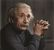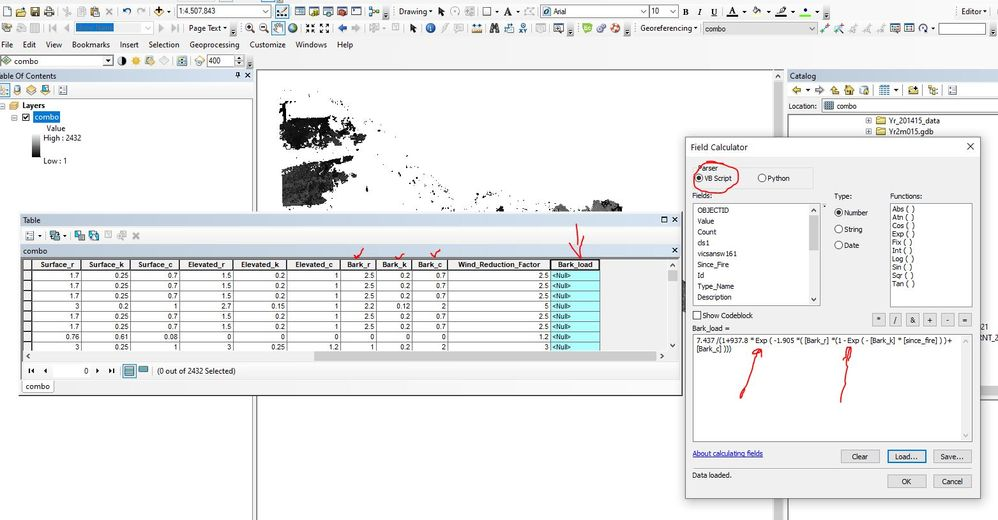# arcpy calculatefield expression codeblock exponential

786
7
03-16-2021 07:28 PMNew Contributor II

Hello colleagues

I am trying to use a formula in python. It is a raster dataset and I am trying to calculate a field based on an equation.

Here is the code

====================

expression = "t_RF(!Bark_r!, !Bark_k!, !Bark_c!, !since_fire!)"

codeblock = """

def t_RF(Bark_r, Bark_k, Bark_c, since_fire):

if since_fire >= 0:

return 7.437 /(1+937.8 * Exp ( -1.905 *( Bark_r *(1 - Exp ( - Bark_k * since_fire ) )+ Bark_c )))

else:

return 999 """

arcpy.CalculateField_management(inTable, fieldName, expression, "PYTHON_9.3", codeblock)

====================

End code

Error message starts

====================

Traceback (most recent call last):

File "C:\Data\A3_Large_projects\Fuelloadmodels\Python_script\Fuelhazard.py", line 126, in <module>

arcpy.CalculateField_management(inTable, fieldName, expression, "PYTHON_9.3", codeblock)

File "C:\Program Files (x86)\ArcGIS\Desktop10.5\ArcPy\arcpy\management.py", line 3661, in CalculateField

raise e

ExecuteError: ERROR 000539: Error running expression: t_RF(2.5, 0.2, 0.7, 1)

Traceback (most recent call last):

File "<expression>", line 1, in <module>

File "<string>", line 4, in t_RF

NameError: global name 'Exp' is not defined

Failed to execute (CalculateField).

====================

End error message

I am relatively new in python. Trying to understand the error message. Will appreciate any help.

Thanks

7 RepliesbyMVP Esteemed Contributor

You didn't import the spatial analyst

Exp (Spatial Analyst)—ArcGIS Pro | Documentation

from arcpy.sa import Exp

And I hope your code block is formatted properly because you copy paste won't work

Code formatting ... the Community Version - Esri Community

... sort of retired...byMVP Esteemed Contributor

This is a raster dataset? You are using Calculate Field, it so sounds like !Bark_r!, !Bark_k!, !Bark_c!, !since_fire!) are all fields in a raster table. (Combine output?)

If that's the case, math.exp should be used not Exp here.

``````>>> import math
>>> math.exp(1)
2.718281828459045``````

However, if I am wrong and these are rasters, not fields in a table, @DanPatterson is correct and you should be doing something like

``````from arcpy.sa import *
outraster = 7.437 /(1+937.8 * Exp ( -1.905 * Bark_k ...``````New Contributor IIThanks, @curtvprice for your help.

Yes, it is a raster dataset name "combo" and those are the field names from the raster table. I trying to calculate a field called "Bark_load" based on the following equation.

VB

7.437 /(1+937.8 * Exp ( -1.905 *( Bark_r *(1 - Exp ( - Bark_k * since_fire ) )+ Bark_c )))

Python

7.437 /(1+937.8 * math.exp(-1.905 *( !Bark_r! *(1 - math.exp(- !Bark_k! * !Since_Fire! )) + !Bark_c! )))

please note: exp is exponential function not Exp (Spatial Analyst)

I have a modelbuilder and this equation works there as VB and Python.

Thanks for any further help.byMVP Esteemed Contributor

Here is how that works with the tool:

``````inTable = "C:/Data/A3_Large_projects/Fuelloadmodels/Data/GDB/Yr2021.gdb/combo"
expr = "t_RF(!Bark_r!, !Bark_k, !Bark_c!, !since_fire!)"
cblock = """
def t_RF(rr, kk, cc, sfire):
import math
if sfire >= 0:
return (
7.437 /
( 1 + 937.8 * math.exp(-1.905 *
(rr * (1 - math.exp(-kk * sfire)) + cc )))
)
else:
return 999 """
arcpy.CalculateField_management(inTable, fieldName, expr, "PYTHON_9.3", cblock)``````

I should mention that arcpy.da.UpdateCursor is a more common way to do this with complex field calculations in Python (less overhead):

``````inTable = "C:/Data/A3_Large_projects/Fuelloadmodels/Data/GDB/Yr2021.gdb/combo"
def t_RF(rr, kk, cc, sfire):
import math
if sfire >= 0:
return (
7.437 /
( 1 + 937.8 * math.exp(-1.905 *
(rr * (1 - math.exp(-kk * sfire)) + cc )))
)
else:
return 999
with arcpy.da.UpdateCursor(inTable,
["Bark_load", "Bark_r", "Bark_k", "Bark_c","since_fire"]) as rows:
for row in rows:
bark_load, rr, kk, cc, sfire = row
row = t_RF(rr, kk, cc, sfire)
rows.updateRow(row)``````byMVP Esteemed Contributor

exponent

``7.437 /(1+937.8**(-1.905 * Bark_k ...``

exponents in python are **

2**4
16

... sort of retired...byMVP Esteemed Contributor

Dan: the VB function and Raster tool Exp is "e to the value" not  ^ (VB) or ** (Python)byMVP Esteemed Contributor

grief... too many e E eE Exp EXP 's ;)

... sort of retired...